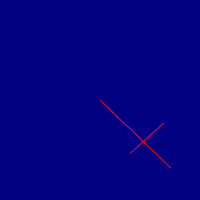Friday, 11 September 2015

Line-Line intersection test

I once did some programming that required mathematical line-line intersections, and it's really something that can't always be easily found ready-made. The mathematical formulae and "theory" are readily available, but are not easily converted to practical situations.

Below I have a Processing sketch that includes the function intersection which compares two lines. Copy/paste the text below into a processing sketch window and go. Everything else outside the function is just to facilitate the demo sketch. Moving the mouse pointer around moves the other line, whereas the other line moves randomly.

It's based on a very old program so it might not be up to scratch. My previous work also returned the intersection coordinate (what I was really after), so this is clearly a simpler case.

There's always the problem that two parallel lines, even if they overlap visibly, coordinate-wise they may not intersect mathematically. For example, lines 0,0-100,0 and 50,0-150,0 do not intersect. The program below does not solve this case. I've supposed that making multiple intersection tests with suitably "jiggled" coordinates might fix this problem for some practical purposes.Not intersecting!Intersecting!

float [] xp=new float;
float [] yp=new float;
float [] xv=new float;
float [] yv=new float;

boolean intersection(float X1,float Y1,float X2,float Y2,float X3,float Y3,float X4,float Y4)
{

//Tests intersection between lines x1,y1-x2,y2 and x3,y3-x4,y4
//Not sure where the original formulas are from.

//The function has the practical problem that parallel lines do not intersect.
//This may be circumvented by "jiggling" the line coordinates just a bit and making two intersection tests, not implemented here

//My original function returned the intersection coordinate, this is a simplification

float ua,ub,denom,numer;

//not sure if the following are needed really

if(X3>X2&&X4>X2&&X3>X1&&X4>X1){return false;}
if(X3<X2&&X4<X2&&X3<X1&&X4<X1){return false;}
if(Y3>Y2&&Y4>Y2&&Y3>Y1&&Y4>Y1){return false;}
if(Y3<Y2&&Y4<Y2&&Y3<Y1&&Y4<Y1){return false;}

// the proper calculations

numer=((X4-X3)*(Y1-Y3)-(Y4-Y3)*(X1-X3));
denom=((Y4-Y3)*(X2-X1)-(X4-X3)*(Y2-Y1));

if(denom==0){return false;}

ua=(numer/denom);
numer=((X2-X1)*(Y1-Y3)-(Y2-Y1)*(X1-X3));
ub=(numer/denom);

if(ua==0&&ub==0){return true;}
if(ub>0&&ub<=1&&ua>0&&ua<=1){return true;}

return false;
}

void draw_lines(float xa1,float ya1,float xa2,float ya2,float xb1,float yb1,float xb2,float yb2)
{

if(intersection(xa1,ya1,xa2,ya2,xb1,yb1,xb2,yb2)==true){
stroke(255,0,0);line(xa1,ya1,xa2,ya2);line(xb1,yb1,xb2,yb2);
}else
{
stroke(0,255,0);line(xa1,ya1,xa2,ya2);line(xb1,yb1,xb2,yb2);
}
}

void setup()
{
size(512,512);
strokeWeight(2);
xp=16;yp=16;xp=64;yp=64;
xv=2;yv=1;
xv=-3;yv=-1;
xp=width/2;yp=height/2;xp=width/2;yp=height/2;
}

void mousePressed()
{
xp=mouseX;yp=mouseY;
}

void draw()
{
background(0,0,128);
draw_lines(xp,yp,xp,yp,xp,yp,xp,yp);
for(int i=0;i<=7;i++){
xp[i]=xp[i]+xv[i];
yp[i]=yp[i]+yv[i];
if(xp[i]>=width){xv[i]=-(1+random(2));}
if(xp[i]<=0){xv[i]=1+random(2);}
if(yp[i]>=height){yv[i]=-(1+random(2));}
if(yp[i]<=0){yv[i]=1+random(2);}
}

xp=mouseX;
yp=mouseY;
}